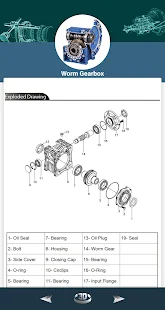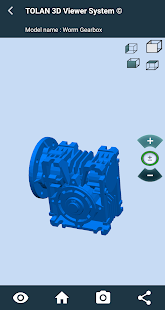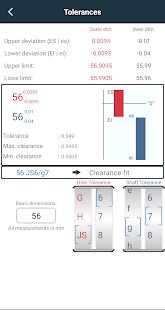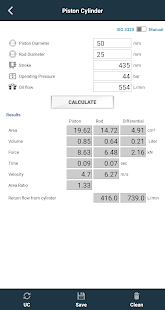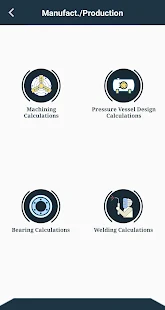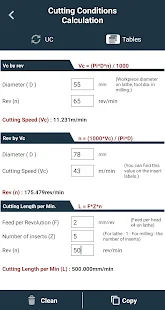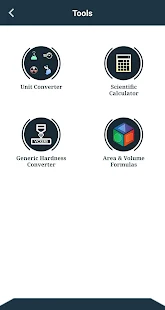Descriptions : This mechanical engineering app is an all-in-one app for all mechanical engineering requirements. It contains various important tools for mechanical engineering.
Features :

+ Detailed heat loss calculations
+ Calculations for natural gas installations
+ Ventilation calculations
+ Other calculations (pipe diameter, circulation pump and Hydrofor calculations)
+ Piston cylinder calculations
+ Pump calculations
+ Battery calculations
+ Pipe calculations
+ Engine calculations
+ Temperature and pressure loss calculations
+ Air consumption calculations
+ Force calculations
+ Calculation of the cutting conditions
+ Calculations for the design of pressure vessels
+ Warehouse calculations
+ Hardness converter
+ Welding calculation; Welding heat input calculations
+ Reinforced concrete bar (quantity) / (area) comparison calculation.
+ Tolerance and fitting calculator and geometric tolerances
+ Theoretical calculations (Bernoulli, Young's module, differential pressure calculation, etc.)
+ A total of 15 mechanical property values ​​for 73 materials can be easily accessed at any time.
+ Material information: iron-carbon phase diagram, elastic-plastic diagram. Stress-strain diag.
+ Convert between hundreds of units in 26 different categories with the unit converter.
+ You can save the results of your calculations in PDF format and view them later.

Required Android Versions : Lollipop [5.0–5.0.2] - Marshmallow [6.0 - 6.0.1] - Nougat [7.0 – 7.1.1] - Oreo [8.0-8.1]